# Simulated Land Scenes for Synthetic Aperture Radar Image Formation

A synthetic aperture radar (SAR) system uses platform motion to mimic a longer aperture to improve cross-range resolution. SAR data is often collected using platforms such as aircraft, spacecraft, or vehicle. Due to the movement of the platform, the data returned from the reflections of targets appears unfocused, looking at times like random noise. In SAR systems, essential information resides in the amplitude and phase of the received data. Phase-dependent processing is critical to the formation of focused images.

In this example, you will simulate an L-band remote-sensing SAR system. The simulation includes generating IQ signals from a scenario containing three targets and a wooded-hills land surface. The returned data will be processed using the range migration focusing algorithm.

For more information on key SAR concepts, see the Stripmap Synthetic Aperture Radar (SAR) Image Formation example.

### Generate Simulated Terrain

Generate and plot a random height map for the land surface. The height map will be representative of a hilly scene with peaks up to about 200 m. It is important to ensure that the terrain resolution is less than the resolution of the imaging system. Based on the configuration of the helper function, the resolution of the map will be a little more than 1 meter in the X dimension and about 1.6 in the Y dimension. To increase the resolution of the generated map, increase the number of iterations. The roughness factor is often set to a value of 2. Smaller values result in rougher terrain, and larger values result in smoother terrain.

```% Initialize random number generator rng(2021) % Create terrain xLimits = [900 1200]; % x-axis limits of terrain (m) yLimits = [-200 200]; % y-axis limits of terrain (m) roughnessFactor = 1.75; % Roughness factor initialHgt = 0; % Initial height (m) initialPerturb = 200; % Overall height of map (m) numIter = 8; % Number of iterations [x,y,A] = helperRandomTerrainGenerator(roughnessFactor,initialHgt, .... initialPerturb,xLimits(1),xLimits(2), ... yLimits(1),yLimits(2),numIter); A(A < 0) = 0; % Fill-in areas below 0 xvec = x(1,:); yvec = y(:,1); resMapX = mean(diff(xvec))```
```resMapX = 1.1719 ```
`resMapY = mean(diff(yvec))`
```resMapY = 1.5625 ```
```% Plot simulated terrain helperPlotSimulatedTerrain(xvec,yvec,A)```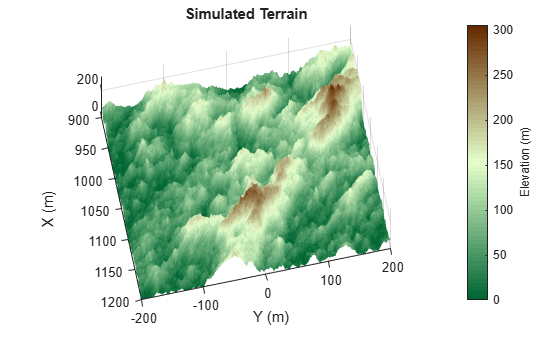### Specify the SAR System and Scenario

Define an L-band SAR imaging system with a range resolution approximately equal to 5 m. This system is mounted on an airborne platform flying at an altitude of 1000 meters. Verify the range resolution is as expected using the `bw2rangeres` function.

```% Define key radar parameters freq = 1e9; % Carrier frequency (Hz) [lambda,c] = freq2wavelen(freq); % Wavelength (m) bw = 30e6; % Signal bandwidth (Hz) fs = 60e6; % Sampling frequency (Hz) tpd = 3e-6; % Pulse width (sec) % Verify that the range resolution is as expected bw2rangeres(bw)```
```ans = 4.9965 ```

Specify the antenna aperture as 6 meters. Set the squint angle to 0 degrees for broadside operation.

```% Antenna properties aperture = 6; % Aperture area (m) sqa = 0; % Squint angle (deg)```

Set the platform velocity to 100 m/s and configure the platform geometry. The synthetic aperture length is 100 m as calculated by `sarlen`.

```% Platform properties v = 100; % Speed of the platform (m/s) dur = 1; % Duration of flight (s) rdrhgt = 1000; % Height of platform (m) rdrpos1 = [0 0 rdrhgt]; % Start position of the radar (m) rdrvel = [0 v 0]; % Radar plaform velocity rdrpos2 = rdrvel*dur + rdrpos1; % End position of the radar (m) len = sarlen(v,dur) % Synthetic aperture length (m)```
```len = 100 ```

Define the targets. The targets in this example are stationary and are intended to represent calibration targets. Set the target heights to 110 meters, relative to the surface. This height was selected such that targets may be occluded due to the hilly terrain.

```% Configure the target platforms in x and y targetpos = [1000,len/2,0;1020,len/2,0;1040,len/2,0]; % Target positions (m) tgthgts = 110*ones(1,3); % Target height (m) for it = 1:3 % Set target height relative to terrain [~,idxX] = min(abs(targetpos(it,1) - xvec)); [~,idxY] = min(abs(targetpos(it,2) - yvec)); tgthgts(it) = tgthgts(it) + A(idxX,idxY); targetpos(it,3) = tgthgts(it); end```

Next, set the reference slant range, which is used in subsequent processing steps such as determining appropriate pointing angles for the radar antenna. Calculate the cross-range resolutions using the `sarazres` function. Based on the geometry and radar settings, the cross-range resolution is about 2 meters.

```% Set the reference slant range for the cross-range processing rc = sqrt((rdrhgt - mean(tgthgts))^2 + (mean(targetpos(:,1)))^2); % Antenna orientation depang = depressionang(rdrhgt,rc,'Flat','TargetHeight',mean(tgthgts)); % Depression angle (deg) grazang = depang; % Grazing angle (deg) % Azimuth resolution azResolution = sarazres(rc,lambda,len) % Cross-range resolution (m)```
```azResolution = 1.9572 ```

Then, determine an appropriate pulse repetition frequency (PRF) for the SAR system. In a SAR system, the PRF has dual implications. The PRF not only determines the maximum unambiguous range but also serves as the sampling frequency in the cross-range direction. If the PRF is too low, the pulses are long in duration, resulting in fewer pulses illuminating a particular region. If the PRF is too high, the cross-range sampling is achieved but at the cost of reduced maximum range. The `sarprfbounds` function suggests maximum and minimum PRF bounds.

```% Determine PRF bounds [swlen,swwidth] = aperture2swath(rc,lambda,aperture,grazang); [prfmin,prfmax] = sarprfbounds(v,azResolution,swlen,grazang)```
```prfmin = 51.0925 ```
```prfmax = 6.4742e+05 ```

For this example, set the PRF to 500 Hz.

```% Select a PRF within the PRF bounds prf = 500; % Pulse repetition frequency (Hz)```

Now that the parameters for the radar and targets are defined. Set up a radar scene using `radarScenario`. Add the radar platform and targets to the scene with `platform`. Set the target radar cross section (RCS) to 5 dBsm, and plot the scene.

```% Create a radar scenario scene = radarScenario('UpdateRate',prf,'IsEarthCentered',false,'StopTime',dur); % Add platforms to the scene using the configurations previously defined rdrplat = platform(scene,'Trajectory',kinematicTrajectory('Position',rdrpos1,'Velocity',[0 v 0])); % Add target platforms rcs = rcsSignature('Pattern',5); for it = 1:3 platform(scene,'Position',targetpos(it,:),'Signatures',{rcs}); end % Plot ground truth helperPlotGroundTruth(xvec,yvec,A,rdrpos1,rdrpos2,targetpos)```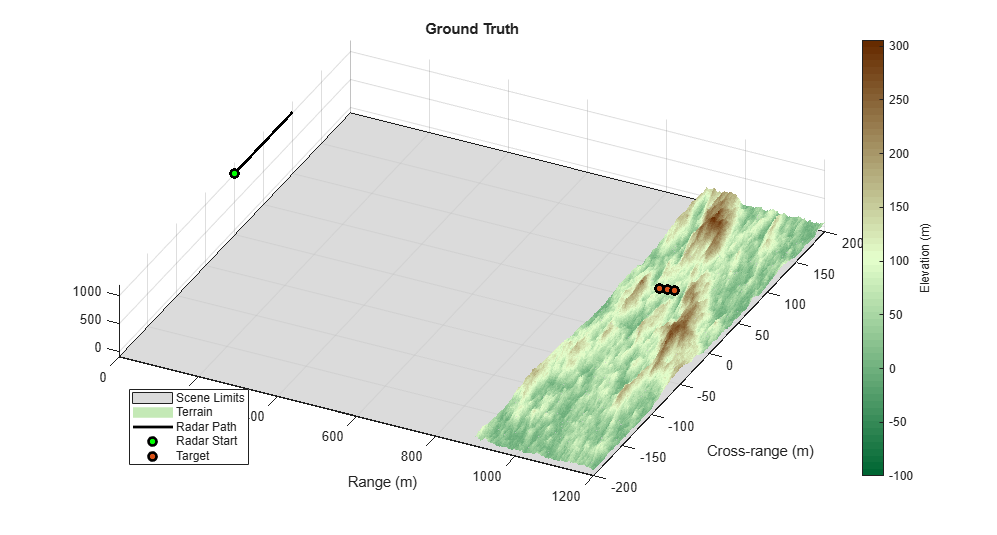Note that the terrain generated has been limited in range and cross-range to the expected beam location. This is to conserve memory, as well as to speed up the simulation.

### Define the Land Surface Reflectivity

Now that the radar scenario and its platforms are set, define the land surface. First, select a reflectivity model. Radar Toolbox™ offers 7 different reflectivity models covering a wide range of frequencies, grazing angles, and land types. The asterisk denotes the default model. Type `help` `landreflectivity` or `doc` `landreflectivity` in the Command Window for more information on usage and applicable grazing angles for each model. The land reflectivity models are as follows.

• APL: Mathematical model supporting low to high grazing angles over frequencies in the range from 1 to 100 GHz. Land types supported are urban, high-relief, and low-relief.

• Barton*: Mathematical model supporting medium grazing angles over frequencies in the range from 1 to 10 GHz. Land types supported are rugged mountains, mountains, metropolitan, urban, wooded hills, rolling hills, woods, farm, desert, flatland, and smooth. This is the default model.

• Billingsley: Empirical model valid for low grazing angles over frequencies from 3 MHz to 12 GHz. Land types supported are low-relief rural, low-relief forest, farm, desert, marsh, grassland, high-relief rural, high-relief forest, mountains, urban, low-relief urban.

• GIT: Semi-empirical model for medium grazing angles over frequencies in the range from 3 to 15 GHz. Land types supported are soil, grass, tall grass, trees, and urban.

• Morchin: Mathematical model for high grazing angles over frequencies in the range from 300 MHz to 8 GHz. Land types supported are desert, farm, woods, and mountains.

• Nathanson: Empirical model for low to medium grazing angles over frequencies in the range from 1 to 36 GHz. Land types supported are desert, farm, woods, jungle, rolling hills, and urban.

• Ulaby-Dobson: Semi-empirical model for low to medium grazing angles over frequencies in the range from 1 to 18 GHz. Land types supported are soil, grass, shrubs, and short vegetation.

For this example, use the default Barton model, since it has such a large number of land types. For terrain values above 100 meters, assign reflectivity values for wooded hills. Otherwise, set reflectivity values to woods. Plot the reflectivity map to see the assignments

```% Specify custom reflectivity map grazTable = 20:0.1:60; freqTable = [1e9 10e9]; numSurfaces = 2; reflectivityLayers = zeros(numel(grazTable),numel(freqTable),numSurfaces); reflectivityLayers(:,:,1) = landreflectivity('Woods', ... grazTable,freqTable); reflectivityLayers(:,:,2) = landreflectivity('WoodedHills', ... grazTable,freqTable); reflectivityType = ones(size(A)); reflectivityType(A > 100) = 2; % Plot custom reflectivity map helperPlotReflectivityMap(xvec,yvec,A,reflectivityType,rdrpos1,rdrpos2,targetpos)```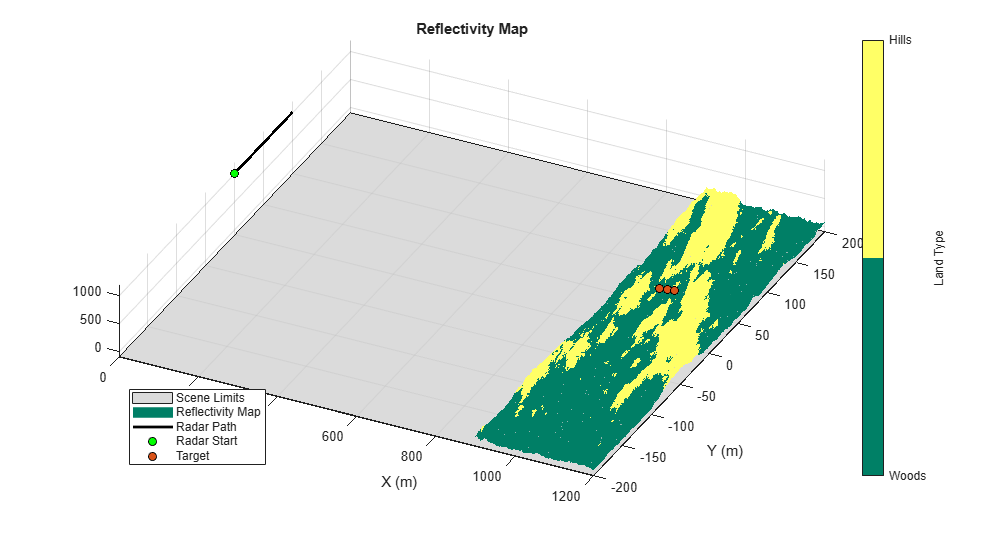Next, compose the custom reflectivity map for use by radarScenario using `surfaceReflectivity`. Set the `Speckle` property to `Rayleigh`. The `surfaceReflectivity` objects model speckle as an uncorrelated multiplicative factor as

$\mathit{I}=\sigma \cdot \mathit{n}$,

where $\sigma$ is the surface reflectivity and $\mathit{n}$ is an independent and identically distributed (IID) random value that is often defined as having unity mean noise. Common speckle models include Weibull, Rayleigh, and lognormal, which are all options provided by `surfaceReflectivity`. You can tailor the speckle with the distribution's corresponding properties. For a Rayleigh distribution, you can modify the `SpeckleScale` property.

```reflectivityMap = surfaceReflectivity('Custom','Frequency',freqTable, ... 'GrazingAngle',grazTable,'Reflectivity',reflectivityLayers, ... 'Speckle','Rayleigh')```
```reflectivityMap = surfaceReflectivityCustom with properties: Reflectivity: [401x2x2 double] Frequency: [1.0000e+09 1.0000e+10] GrazingAngle: [20 20.1000 20.2000 20.3000 20.4000 20.5000 20.6000 ... ] Speckle: 'Rayleigh' SpeckleScale: 1.1284 ```

Add a land surface to the radar scenario using `landSurface`. Assign the random height map previously generated and the reflectivity map to the land surface.

```% Add land surface to scene s = landSurface(scene,'Terrain',A,'Boundary',[xLimits;yLimits], ... 'RadarReflectivity',reflectivityMap, ... 'ReflectivityMap',reflectivityType);```

In this section, configure the radar system properties. Define the antenna and the transmitted linear frequency modulated (LFM) waveform. Assign the radar sensor to the radar platform.

```% Create a radar looking to the right mountAngles = [0 depang 0]; rdr = radarTransceiver('MountingAngles',mountAngles,'NumRepetitions',1); % Set peak power rdr.Transmitter.PeakPower = 50e3; % Set receiver sample rate and noise figure rdr.Receiver.SampleRate = fs; rdr.Receiver.NoiseFigure = 30; % Define transmit and receive antenna and corresponding parameters antbw = ap2beamwidth(aperture,lambda); ant = phased.SincAntennaElement('FrequencyRange',[1e9 10e9],'Beamwidth',antbw); rdr.TransmitAntenna.Sensor = ant; rdr.TransmitAntenna.OperatingFrequency = freq; rdr.ReceiveAntenna.Sensor = ant; rdr.ReceiveAntenna.OperatingFrequency = freq; antennaGain = aperture2gain(aperture,lambda); rdr.Transmitter.Gain = antennaGain; rdr.Receiver.Gain = antennaGain; % Configure the LFM signal of the radar rdr.Waveform = phased.LinearFMWaveform('SampleRate',fs,'PulseWidth',tpd, ... 'PRF',prf,'SweepBandwidth',bw); matchingCoeff = getMatchedFilter(rdr.Waveform); pulseCompress = phased.RangeResponse('RangeMethod', 'Matched filter', ... 'PropagationSpeed',c,'SampleRate',fs); % Add radar to radar platform rdrplat.Sensors = rdr;```

### Generate the Datacube

Now that the scene and the radar system are defined, generate returns from the land surface with the `clutterGenerator` method. By default, `clutterGenerator` will simulate clutter returns in the mainlobe. For more information about clutter modeling, see Introduction to Radar Scenario Clutter Simulation.

```% Collect clutter returns with the clutterGenerator clutterGenerator(scene,rdr); % Initialize output IQ datacube minSample = 500; % Minimum sample range maxRange = 2500; % Maximum range for IQ collection truncRngSamp = ceil(range2time(maxRange)*fs); % Limit the number of samples T = 1/prf; % Pulse repetition interval (sec) numPulses = dur/T + 1; % Number of pulses raw = zeros(numel(minSample:truncRngSamp),numPulses); % IQ datacube ```

Data is collected with the `receive` method. Either load in the data or simulate the raw SAR returns. If you choose to simulate the IQ, as the IQ data is received, a plot of the raw signal returns is generated. Otherwise, the unprocessed datacube is plotted at once. The raw signal is the collection of pulses transmitted in the cross-range direction. The plot shows the real part of the signal for the three targets and the land surface.

```% Collect IQ ii = 1; hRaw = helperPlotRawIQ(raw,minSample); simulateData = false; % Change to true to simulate IQ if simulateData while advance(scene) %#ok<UNRCH> tmp = receive(scene); % nsamp x 1 raw(:,ii) = tmp{1}(minSample:truncRngSamp); if mod(ii,100) == 0 % Update plot after 100 pulses helperUpdatePlotRawIQ(hRaw,raw); end ii = ii + 1; end else load('rawSAR.mat'); end helperUpdatePlotRawIQ(hRaw,raw);```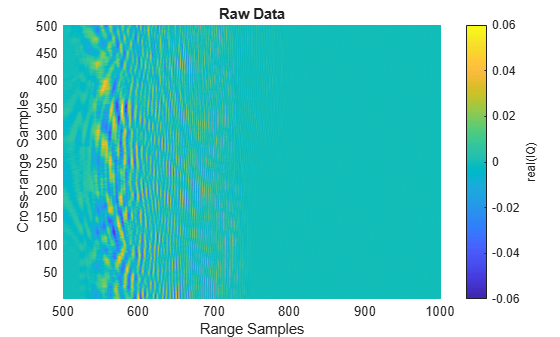As is evident in the fully formed plot, the returns from the targets and the land surface are widely distributed in range and cross-range. Thus, it is difficult to distinguish the individual targets in the raw two-dimensional SAR data. The area where it is evident that returns are present is the mainlobe of the antenna. If you want to image a larger region, a couple of changes you can implement are:

• Increase the altitude of the SAR imaging platform or

• Increase the beamwidth.

### Visualize SAR Data

Focus the image using the range migration algorithm. The range-migration algorithm corrects for the range-azimuth coupling, as well as the azimuth-frequency dependence. The algorithm proceeds as:

1. FFT: First, the algorithm performs a two-dimensional FFT. This transforms the SAR signal into wavenumber space.

2. Matched Filtering: Second, the algorithm focuses the image using a reference signal. This is a bulk focusing stage. The reference signal is computed for a selected range, often the mid-swath range. Targets at the selected range will be correctly focused, but targets away from the reference are only partially focused.

3. Stolt Interpolation: Next is a differential focusing stage that uses Stolt interpolation to focus the remainder of the targets.

4. IFFT: Finally, a two-dimensional IFFT is performed to return the data to the time domain.

Based on the radar waveform, use the `rangeMigrationLFM` function to form the single-look complex (SLC) image and plot the results. After range and cross-range processing, two targets can be distinguished from the background.

```% Generating Single Look Complex image using range migration algorithm slcimg = rangeMigrationLFM(raw,rdr.Waveform,freq,v,rc); helperPlotSLC(slcimg,minSample,fs,v,prf,rdrpos1,targetpos, ... xvec,yvec,A)```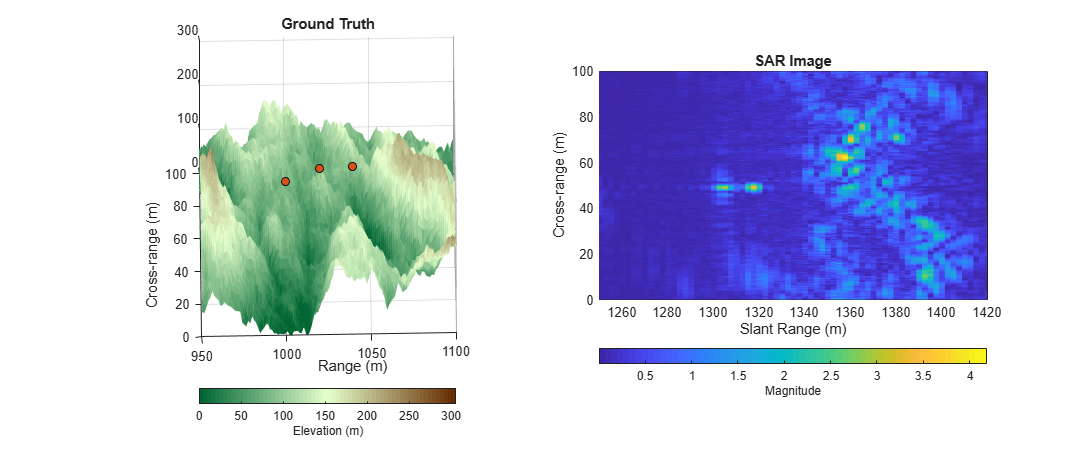SAR images are similar to optical images, but the physics is quite different. Since SAR uses slant range to form images, higher elevation targets appear closer to the radar than lower elevation targets, resulting in higher elevation targets appearing at nearer ranges in the SAR image. This distortion is called layover and is noticeable in the image. Additionally, the actual grazing angles change slightly over the imaged swath, with shallower angles existing at farther ranges and steeper angles at closer ranges. These characteristics among others result in warped images in comparison to the Cartesian ground truth.

The occlusion method helps us to further interpret the results. Using the `occlusion` method on the land surface, determine the visibility of the targets throughout the scenario.

```for it = 1:3 % Determine whether the target was occluded occ = false(1,numPulses); for ip = 1:numPulses rdrpos = rdrpos1 + rdrvel.*1/prf*(ip - 1); occ(ip) = s.occlusion(rdrpos,targetpos(it,:)); end % Translate occlusion values to a visibility status helperGetVisibilityStatus(it,occ) end```
```Target 1 is not visible during the scenario (visible 0% of the data collection). Target 2 is partially visible during the scenario (visible 81% of the data collection). Target 3 is fully visible during the scenario (visible 100% of the data collection). ```

The first target is not visible at all, because it is occluded by the terrain. The second target is only partially visible throughout the collection. This causes missing data in the cross-range dimension, which results in the increased sidelobes and decreased signal power. The third target is fully visible throughout the target collection. It is bright and focused.

### Summary

This example demonstrated how to generate IQ from a stripmap-based SAR scenario with three targets over land terrain. This example showed you how to:

• Configure the SAR radar parameters,

• Build a custom reflectivity map,

```function [x,y,terrain] = helperRandomTerrainGenerator(f,initialHeight, ... initialPerturb,minX,maxX,minY,maxY,numIter) %randTerrainGenerator Generate random terrain % [x,y,terrain] = helperRandomTerrainGenerator(f,initialHeight, ... % initialPerturb,minX,maxX,minY,maxY,seaLevel,numIter) % % Inputs: % - f = A roughness parameter. A factor of 2 is a % typical default. Lower values result in a % rougher terrain; higher values result in a % smoother surface. % - initialHeight = Sets the initial height of the lattice before % the perturbations. % - initialPerturb = Initial perturbation amount. This sets the % overall height of the landscape. % - minX,maxX,minY,maxY = Initial points. Provides some degree of control % over the macro appearance of the landscape. % - numIter = Number of iterations that affects the density % of the mesh that results from the iteration % process. % % Output: % - x = X-dimension mesh grid % - y = Y-dimension mesh grid % - terrain = Two-dimensional array in which each value % represents the height of the terrain at that % point/mesh-cell % Generate random terrain dX = (maxX-minX)/2; dY = (maxY-minY)/2; [x,y] = meshgrid(minX:dX:maxX,minY:dY:maxY); terrain = ones(3,3)*initialHeight; perturb = initialPerturb; for ii = 2:numIter perturb = perturb/f; oldX = x; oldY = y; dX = (maxX-minX)/2^ii; dY = (maxY-minY)/2^ii; [x,y] = meshgrid(minX:dX:maxX,minY:dY:maxY); terrain = griddata(oldX,oldY,terrain,x,y); terrain = terrain + perturb*random('norm',0,1,1+2^ii,1+2^ii); terrain(terrain < 0) = 0; end end function cmap = landColormap(n) %landColormap Colormap for land surfaces % cmap = landColormap(n) % % Inputs: % - n = Number of samples in colormap % % Output: % - cmap = n-by-3 colormap c = hsv2rgb([5/12 1 0.4; 0.25 0.2 1; 5/72 1 0.4]); cmap = zeros(n,3); cmap(:,1) = interp1(1:3,c(:,1),linspace(1,3,n)); cmap(:,2) = interp1(1:3,c(:,2),linspace(1,3,n)); cmap(:,3) = interp1(1:3,c(:,3),linspace(1,3,n)); colormap(cmap); end function helperPlotSimulatedTerrain(xvec,yvec,A) % Plot simulated terrain figure() hS = surf(xvec,yvec,A); hS.EdgeColor = 'none'; hC = colorbar; hC.Label.String = 'Elevation (m)'; landmap = landColormap(64); colormap(landmap); xlabel('X (m)') ylabel('Y (m)') axis equal; title('Simulated Terrain') view([78 78]) drawnow pause(0.25) end function helperPlotGroundTruth(xvec,yvec,A,rdrpos1,rdrpos2,targetpos) % Plot ground truth f = figure('Position',[505 443 997 535]); movegui(f,'center'); % Plot boundary. Set plot boundary much lower than 0 for rendering reasons. hLim = surf([0 1200],[-200 200].',-100*ones(2),'FaceColor',[0.8 0.8 0.8],'FaceAlpha',0.7); hold on; hS = surf(xvec,yvec,A); hS.EdgeColor = 'none'; hC = colorbar; hC.Label.String = 'Elevation (m)'; landmap = landColormap(64); colormap(landmap); hPlatPath = plot3([rdrpos1(1) rdrpos2(1)], ... [rdrpos1(2) rdrpos2(2)],[rdrpos1(3) rdrpos2(3)], ... '-k','LineWidth',2); hPlatStart = plot3(rdrpos1(1),rdrpos1(2),rdrpos1(3), ... 'o','LineWidth',2,'MarkerFaceColor','g','MarkerEdgeColor','k'); hTgt = plot3(targetpos(:,1),targetpos(:,2),targetpos(:,3), ... 'o','LineWidth',2,'MarkerFaceColor',[0.8500 0.3250 0.0980], ... 'MarkerEdgeColor','k'); view([26 75]) xlabel('Range (m)') ylabel('Cross-range (m)') title('Ground Truth') axis tight; zlim([-100 1200]) legend([hLim,hS,hPlatPath,hPlatStart,hTgt], ... {'Scene Limits','Terrain','Radar Path','Radar Start','Target'},'Location','SouthWest') drawnow pause(0.25) end function helperPlotReflectivityMap(xvec,yvec,A,reflectivityType,rdrpos1,rdrpos2,targetpos) % Plot custom reflectivity map f = figure('Position',[505 443 997 535]); movegui(f,'center'); % Plot boundary. Set plot boundary much lower than 0 for rendering reasons. hLim = surf([0 1200],[-200 200].',-100*ones(2),'FaceColor',[0.8 0.8 0.8],'FaceAlpha',0.7); hold on hS = surf(xvec,yvec,A,reflectivityType); hS.EdgeColor = 'none'; hold on; colormap(summer(2)); hC = colorbar; caxis([1 2]); hC.Ticks = [1 2]; hC.TickLabels = {'Woods','Hills'}; hC.Label.String = 'Land Type'; hPlatPath = plot3([rdrpos1(1) rdrpos2(1)],[rdrpos1(2) rdrpos2(2)],[rdrpos1(3) rdrpos2(3)], ... '-k','LineWidth',2); hPlatStart = plot3(rdrpos1(1),rdrpos1(2),rdrpos1(3), ... 'o','MarkerFaceColor','g','MarkerEdgeColor','k'); hTgt = plot3(targetpos(:,1),targetpos(:,2),targetpos(:,3), ... 'o','MarkerFaceColor',[0.8500 0.3250 0.0980],'MarkerEdgeColor','k'); view([26 75]) xlabel('X (m)') ylabel('Y (m)') title('Reflectivity Map') axis tight; zlim([-100 1200]) legend([hLim,hS,hPlatPath,hPlatStart,hTgt], ... {'Scene Limits','Reflectivity Map','Radar Path','Radar Start','Target'},'Location','SouthWest') drawnow pause(0.25) end function hRaw = helperPlotRawIQ(raw,minSample) % Plot real of raw SAR IQ figure() [m,n] = size(raw); hRaw = pcolor(minSample:(m + minSample - 1),1:n,real(raw.')); hRaw.EdgeColor = 'none'; title('Raw Data') xlabel('Range Samples') ylabel('Cross-range Samples') hC = colorbar; caxis([-5e-3 5e-3]) hC.Label.String = 'real(IQ)'; drawnow pause(0.25) end function helperUpdatePlotRawIQ(hRaw,raw) % Update the raw SAR IQ plot hRaw.CData = real(raw.'); caxis([-5e-3 5e-3]); drawnow pause(0.25) end function helperPlotSLC(slcimg,minSample,fs,v,prf,rdrpos1,targetpos, ... xvec,yvec,A) % Plot magnitude of focused SAR image alongside reflectivity map % Cross-range y-vector (m) numPulses = size(slcimg,2); du = v*1/prf; % Cross-range sample spacing (m) dky = 2*pi/(numPulses*du); % ku domain sample spacing (rad/m) dy = 2*pi/(numPulses*dky); % y-domain sample spacing (rad/m) y = dy*(0:(numPulses - 1)) + rdrpos1(2); % Cross-range y-vector (m) % Range vector (m) c = physconst('LightSpeed'); numSamples = size(slcimg,1); samples = minSample:(numSamples + minSample - 1); sampleTime = samples*1/fs; rngVec = time2range(sampleTime(1:end),c); % Initialize figure f = figure('Position',[264 250 1411 535]); movegui(f,'center') tiledlayout(1,2,'TileSpacing','Compact'); % Ground Truth nexttile; hS = surf(xvec,yvec,A); hS.EdgeColor = 'none'; hold on; plot3(targetpos(:,1),targetpos(:,2),targetpos(:,3), ... 'o','MarkerFaceColor',[0.8500 0.3250 0.0980],'MarkerEdgeColor','k'); landmap = landColormap(64); colormap(landmap); hC = colorbar('southoutside'); hC.Label.String = 'Elevation (m)'; view([-1 75]) xlabel('Range (m)') ylabel('Cross-range (m)') title('Ground Truth') axis equal xlim([950 1100]) ylim([0 100]) % SAR Image nexttile; slcimg = abs(slcimg).'; hProc = pcolor(rngVec,y,slcimg); hProc.EdgeColor = 'none'; colormap(hProc.Parent,parula) hC = colorbar('southoutside'); hC.Label.String = 'Magnitude'; xlabel('Slant Range (m)') ylabel('Cross-range (m)') title('SAR Image') axis equal xlim([1250 1420]) ylim([0 100]) drawnow pause(0.25) end function helperGetVisibilityStatus(tgtNum,occ) % Translate occlusion values to a visibility status visibility = {'not','partially','fully'}; if all(occ) idx = 1; elseif any(occ) idx = 2; else idx = 3; end visString = visibility{idx}; pctCollect = sum(double(~occ))./numel(occ)*100; fprintf('Target %d is %s visible during the scenario (visible %.0f%% of the data collection).\n', ... tgtNum,visString,pctCollect) drawnow pause(0.25) end```